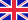•# Conditional probability

Autor: Frederic P. Miller

High Quality Content by WIKIPEDIA articles! Conditional probability is the probability of some event A, given the occurrence of some other event B. Conditional probability is written P(A|B), and is read "the probability of A, given B". Joint probability... Viac o knihe

Na objednávku, dodanie 2-4 týždne

42.84 €

bežná cena: 50.40 €

## O knihe

High Quality Content by WIKIPEDIA articles! Conditional probability is the probability of some event A, given the occurrence of some other event B. Conditional probability is written P(A|B), and is read "the probability of A, given B". Joint probability is the probability of two events in conjunction. That is, it is the probability of both events together. Marginal probability is then the unconditional probability P(A) of the event A; that is, the probability of A, regardless of whether event B did or did not occur. If B can be thought of as the event of a random variable X having a given outcome, the marginal probability of A can be obtained by summing (or integrating, more generally) the joint probabilities over all outcomes for X. This is called marginalization. In these definitions, note that there need not be a causal or temporal relation between A and B. A may precede B or vice versa or they may happen at the same time.

• Vydavateľstvo: Alphascript Publishing
• Rok vydania: 2009
• Formát: Paperback
• Rozmer: 220 x 150 mm
• Jazyk: Anglický jazyk
• ISBN: 9786130246006

Generuje redakčný systém BUXUS CMS spoločnosti ui42.